# Chapter 27 Current and Resistance Electric Current The

• Slides: 32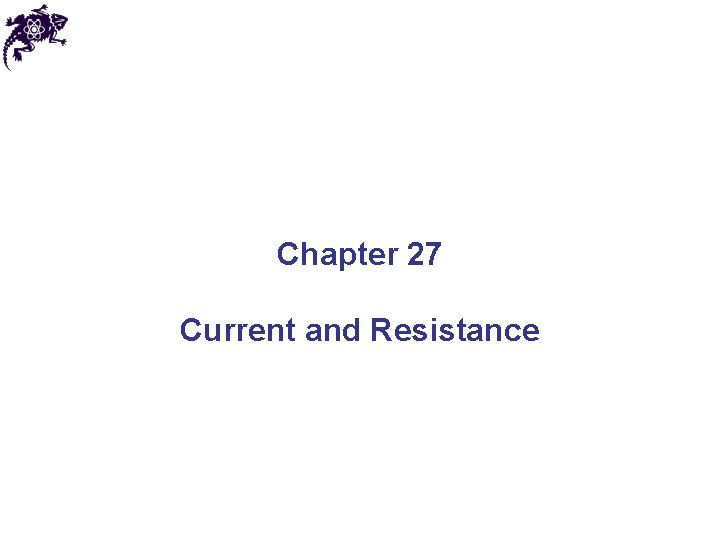Chapter 27 Current and ResistanceElectric Current • The electric current I is the rate of flow of charge through some region of space • The SI unit of current is Ampere (A): 1 A = 1 C/s • Let us look at the charges flowing perpendicularly to a surface of area A • The average current: • The instanteneous current: André-Marie Ampère 1775 – 1836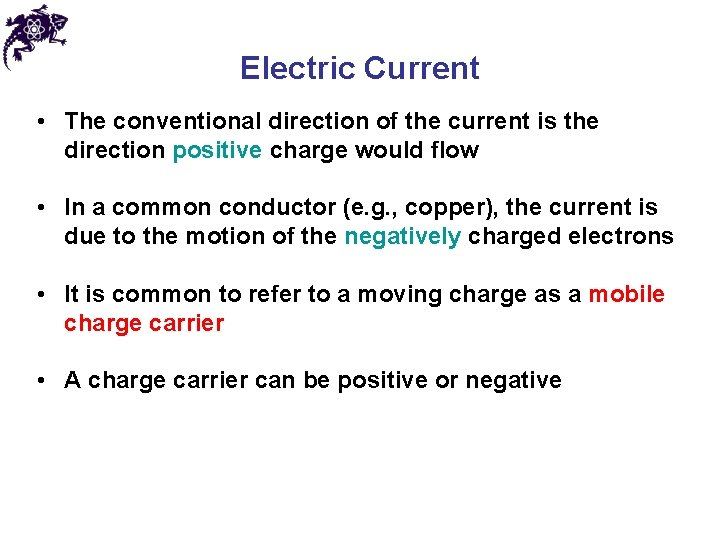Electric Current • The conventional direction of the current is the direction positive charge would flow • In a common conductor (e. g. , copper), the current is due to the motion of the negatively charged electrons • It is common to refer to a moving charge as a mobile charge carrier • A charge carrier can be positive or negative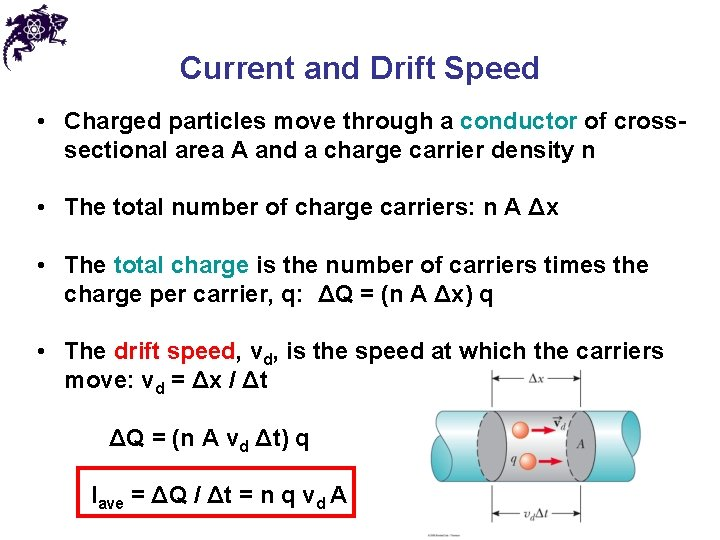Current and Drift Speed • Charged particles move through a conductor of crosssectional area A and a charge carrier density n • The total number of charge carriers: n A Δx • The total charge is the number of carriers times the charge per carrier, q: ΔQ = (n A Δx) q • The drift speed, vd, is the speed at which the carriers move: vd = Δx / Δt ΔQ = (n A vd Δt) q Iave = ΔQ / Δt = n q vd ACurrent and Drift Speed • If the conductor is isolated, the electrons undergo random motion (due to collisions with the atoms) • When an electric field is set up in the conductor, it creates an electric force on the electrons and hence a current • The zigzag line represents the motion of charge carrier in a conductorCurrent and Drift Speed • The drift speed is much smaller than the average speed between collisions • When a circuit is completed, the electric field travels with a speed close to the speed of light • Therefore, although the drift speed is on the order of 10 -4 m/s the effect of the electric field is felt on the order of 108 m/s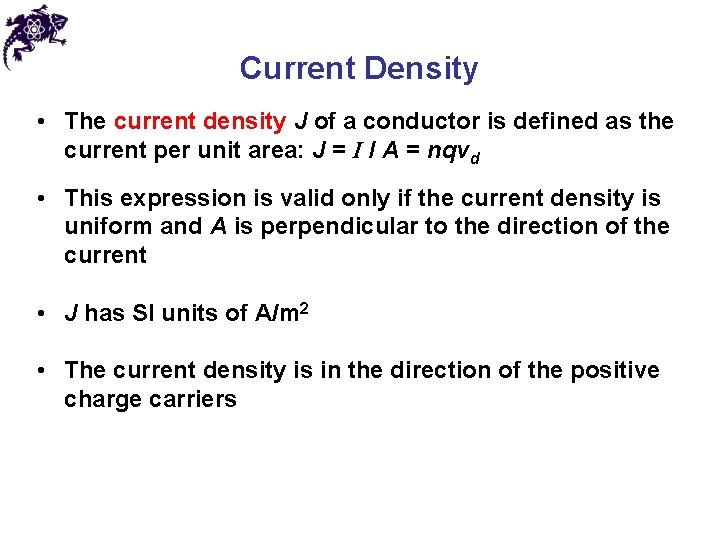Current Density • The current density J of a conductor is defined as the current per unit area: J = I / A = nqvd • This expression is valid only if the current density is uniform and A is perpendicular to the direction of the current • J has SI units of A/m 2 • The current density is in the direction of the positive charge carriers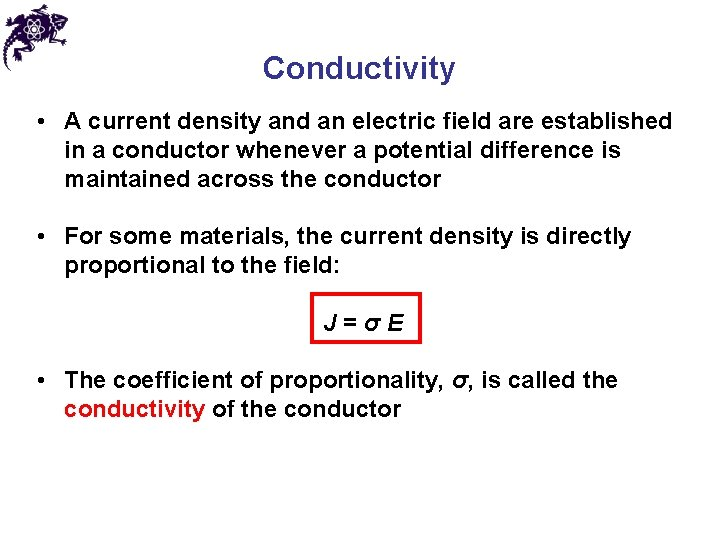Conductivity • A current density and an electric field are established in a conductor whenever a potential difference is maintained across the conductor • For some materials, the current density is directly proportional to the field: J=σE • The coefficient of proportionality, σ, is called the conductivity of the conductor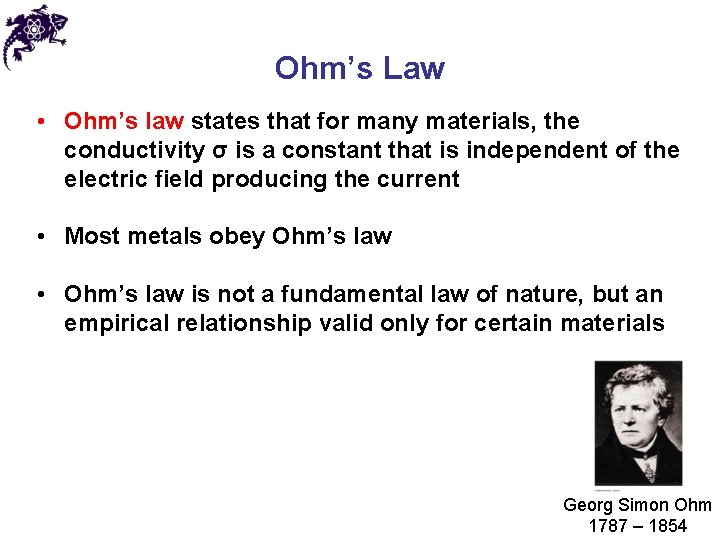Ohm’s Law • Ohm’s law states that for many materials, the conductivity σ is a constant that is independent of the electric field producing the current • Most metals obey Ohm’s law • Ohm’s law is not a fundamental law of nature, but an empirical relationship valid only for certain materials Georg Simon Ohm 1787 – 1854Resistance • In a conductor, the voltage applied across the ends of the conductor is proportional to the current through the conductor • The constant of proportionality is the resistance of the conductor – it arises due to collisions between the electrons carrying the current with the fixed atoms inside the conductor • SI unit of resistance is ohm (Ω): 1 Ω = 1 V / A Georg Simon Ohm 1787 – 1854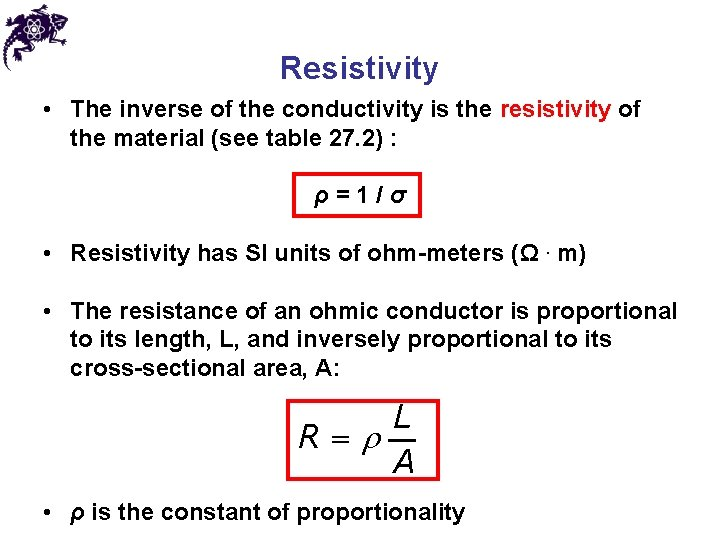Resistivity • The inverse of the conductivity is the resistivity of the material (see table 27. 2) : ρ=1/σ • Resistivity has SI units of ohm-meters (Ω. m) • The resistance of an ohmic conductor is proportional to its length, L, and inversely proportional to its cross-sectional area, A: L R=r A • ρ is the constant of proportionalityOhmic and Nonohmic Materials • Materials that obey Ohm’s Law are said to be ohmic (the relationship between current and voltage is linear, and the resistance is constant over a wide range of voltages) • Not all materials follow Ohm’s law • Materials that do not obey Ohm’s law are said to be nonohmic Non-ohmicResistance and Resistivity, Summary • Every ohmic material has a characteristic resistivity that depends on the properties of the material and on temperature, i. e. , resistivity is a property of substances • The resistance of a material depends on its geometry and its resistivity, i. e. , resistance is a property of an object • An ideal conductor would have zero resistivity • An ideal insulator would have infinite resistivityChapter 27 Problem 13 Suppose that you wish to fabricate a uniform wire out of 1. 00 g of copper. If the wire is to have a resistance R = 0. 500 Ω, and if all of the copper is to be used, what will be (a) the length and (b) the diameter of the wire?A Model for Electrical Conduction • Treat a conductor as a regular array of atoms plus a collection of free electrons – conduction electrons • In the absence of an electric field, the motion of the conduction electrons is random, and their speed is on the order of 106 m/s • When an electric field is applied, the conduction electrons are given a drift velocity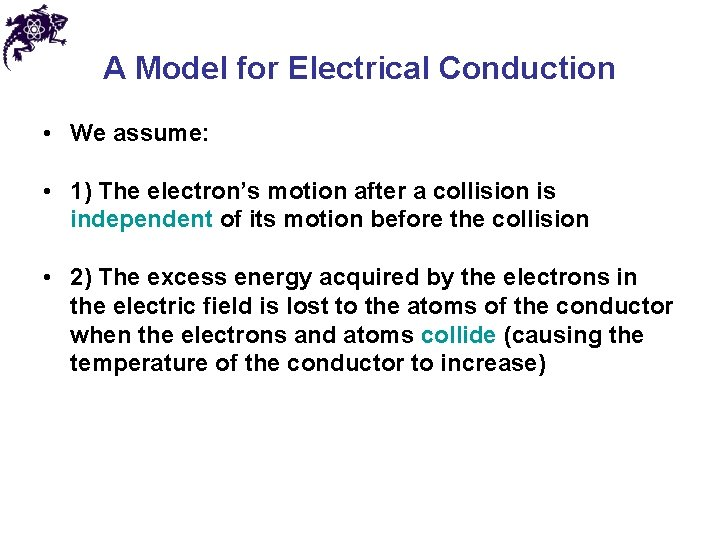A Model for Electrical Conduction • We assume: • 1) The electron’s motion after a collision is independent of its motion before the collision • 2) The excess energy acquired by the electrons in the electric field is lost to the atoms of the conductor when the electrons and atoms collide (causing the temperature of the conductor to increase)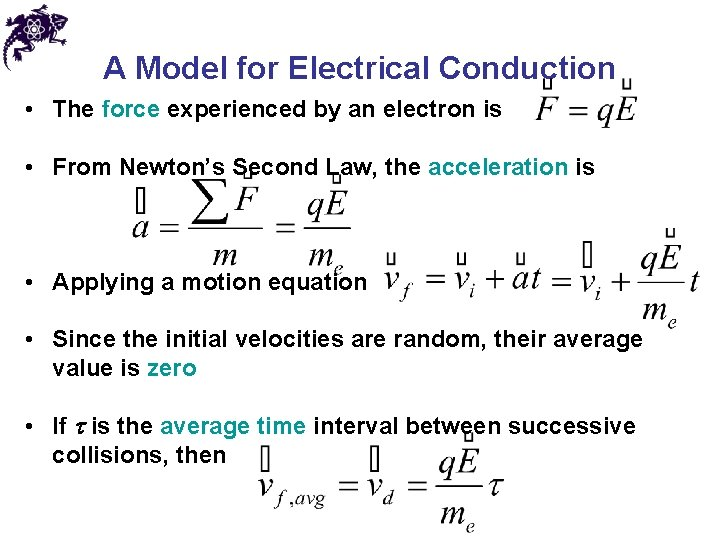A Model for Electrical Conduction • The force experienced by an electron is • From Newton’s Second Law, the acceleration is • Applying a motion equation • Since the initial velocities are random, their average value is zero • If t is the average time interval between successive collisions, then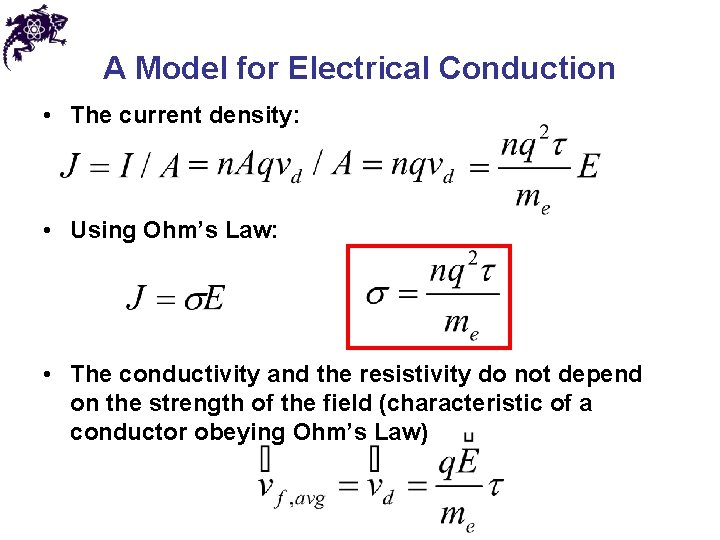A Model for Electrical Conduction • The current density: • Using Ohm’s Law: • The conductivity and the resistivity do not depend on the strength of the field (characteristic of a conductor obeying Ohm’s Law)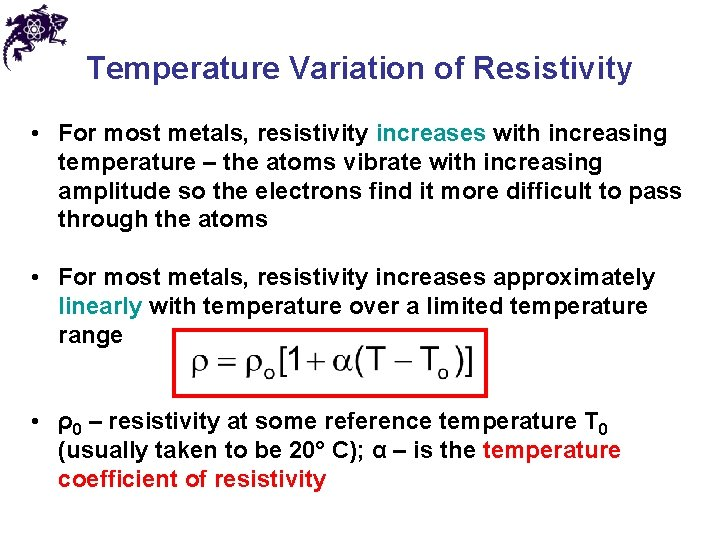Temperature Variation of Resistivity • For most metals, resistivity increases with increasing temperature – the atoms vibrate with increasing amplitude so the electrons find it more difficult to pass through the atoms • For most metals, resistivity increases approximately linearly with temperature over a limited temperature range • ρ0 – resistivity at some reference temperature T 0 (usually taken to be 20° C); α – is the temperature coefficient of resistivityTemperature Variation of Resistance • Since the resistance of a conductor with uniform cross sectional area is proportional to the resistivity, the effect of temperature on resistance is similarChapter 27 Problem 22 An aluminum rod has a resistance of 1. 234 Ω at 20. 0°C. Calculate the resistance of the rod at 120°C by accounting for the changes in both the resistivity and the dimensions of the rod.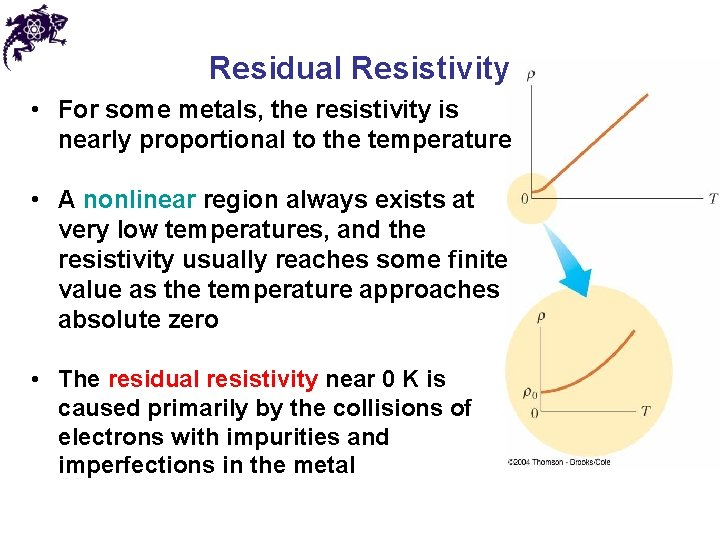Residual Resistivity • For some metals, the resistivity is nearly proportional to the temperature • A nonlinear region always exists at very low temperatures, and the resistivity usually reaches some finite value as the temperature approaches absolute zero • The residual resistivity near 0 K is caused primarily by the collisions of electrons with impurities and imperfections in the metalSuperconductors • Superconductors – a class of materials whose resistances fall to virtually zero below a certain temperature, TC (critical temperature) • The value of TC is sensitive to chemical composition, pressure, and crystalline structure • Once a current is set up in a superconductor, it persists without any applied voltage (since R = 0) • One application is superconducting magnetsSemiconductors • Semiconductors are materials that exhibit a decrease in resistivity with an increase in temperature, i. e. α is negative • The reason – an increase in the density of charge carriers at higher temperatures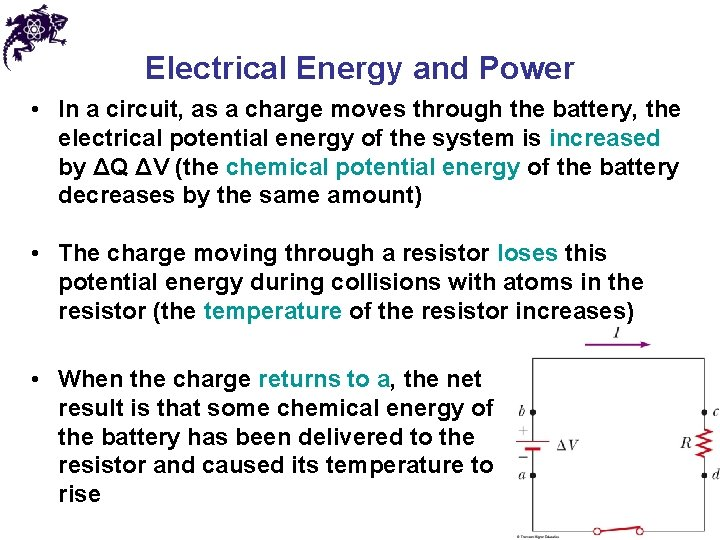Electrical Energy and Power • In a circuit, as a charge moves through the battery, the electrical potential energy of the system is increased by ΔQ ΔV (the chemical potential energy of the battery decreases by the same amount) • The charge moving through a resistor loses this potential energy during collisions with atoms in the resistor (the temperature of the resistor increases) • When the charge returns to a, the net result is that some chemical energy of the battery has been delivered to the resistor and caused its temperature to riseElectrical Energy and Power • The rate at which the energy is lost is the power • From Ohm’s Law, alternate forms of power are • The SI unit of power is Watt (W) (I must be in Amperes, R in ohms and ΔV in Volts) • The unit of energy used by electric companies is the kilowatt-hour (defined in terms of the unit of power and the amount of time it is supplied): 1 k. Wh = 3. 60 x 106 J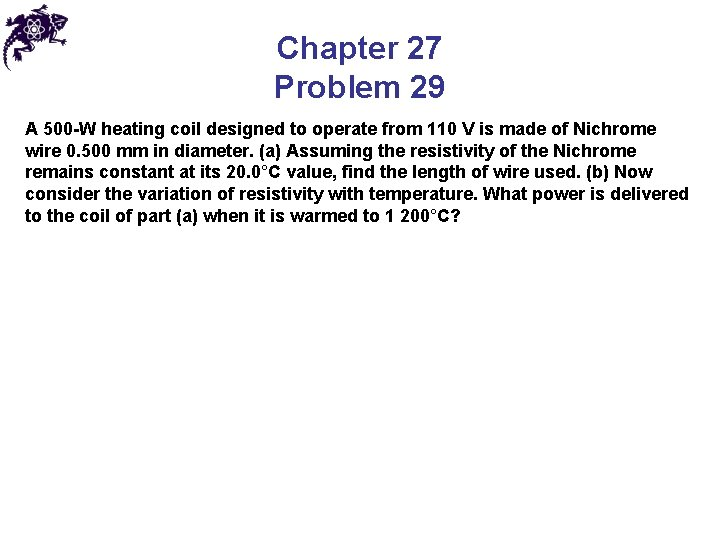Chapter 27 Problem 29 A 500 -W heating coil designed to operate from 110 V is made of Nichrome wire 0. 500 mm in diameter. (a) Assuming the resistivity of the Nichrome remains constant at its 20. 0°C value, find the length of wire used. (b) Now consider the variation of resistivity with temperature. What power is delivered to the coil of part (a) when it is warmed to 1 200°C?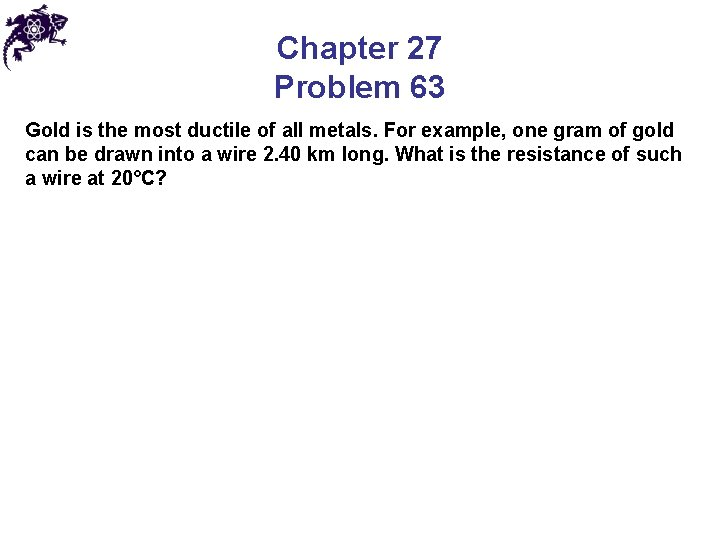Chapter 27 Problem 63 Gold is the most ductile of all metals. For example, one gram of gold can be drawn into a wire 2. 40 km long. What is the resistance of such a wire at 20°C?Answers to Even Numbered Problems Chapter 27: Problem 10 0. 130 mm/sAnswers to Even Numbered Problems Chapter 27: Problem 12 500 m. AAnswers to Even Numbered Problems Chapter 27: Problem 18 1. 44 × 103 °CAnswers to Even Numbered Problems Chapter 27: Problem 44 27. 0 yr